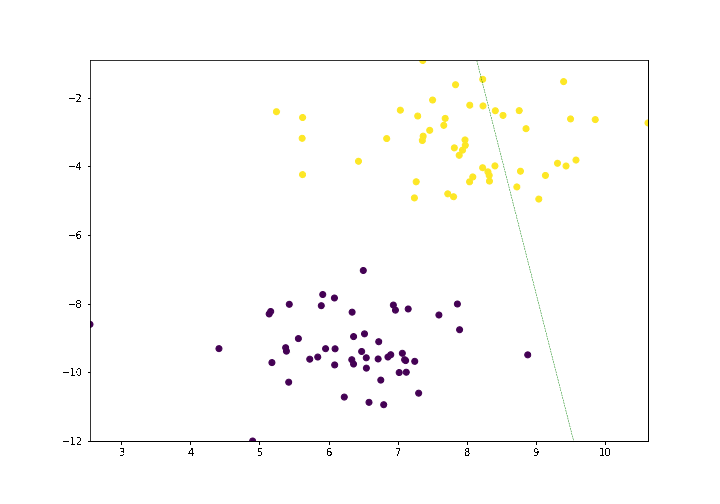# Day 7: Perceptrons from scratch using NumPy.

Perceptron implementation from scratch using NumPy and Matplotlib. Dataset via Scikit Learn.

Jun 07, 2021Perceptron algorithm is a building block of Neural Networks. In this notebook, we implement the Perceptrons in NumPy and plot using matplotlib.

#### Perceptron Algorithm in NumPy and Matplotlib

Perceptron algorithm is a building block of Neural Networks. In this notebook, we implement the Perceptrons in NumPy and plot using matplotlib.

##### Perceptron is denoted as

\begin{aligned} W_{x} + b = \sum_{i=1}^{n} w_{i} + b \\ \end{aligned}

##### Step Function

Above output is passed through this step function

"Yes" if $W_{x} + b \geq 0$ else "No"

##### Assumptions
1. Binary classification dataset.
2. Labels are only 0 and 1.
3. Weights are denoted by W.
4. Labels are denoted by y.
5. Features are denoted by X.
6. Learning rate is denoted by $\alpha$.##### Pseudocode for Perceptron Algorithm Implementation.
Initialize random weights $W_{1}, W_{2}, W_{3} .... W_{n}$.
For every mis-classified point $X_{1}, X_{2}, X_{3} .... X_{n}$.
If prediction=0:
For i=1,2....n:
Change $W_{i} + \alpha*X_{i} where \alpha$ is learning rate
Change $b = b + \alpha$
If prediction=1:
For i=1,2....n:
Change $W_{i} - \alpha*X_{i} where \alpha$ is learning rate
Change $b = b - \alpha$

Wanna jump right to code, check out complete code on Github.

##### Import libraries
import numpy as np
import pandas as pd
from sklearn import datasets

import matplotlib.pyplot as plt

%matplotlib inline
plt.rcParams['figure.figsize'] = [10, 7]
##### Create and Visualize Data
X, y = datasets.make_blobs(n_samples=100, n_features=2, centers=2, cluster_std=1.05, random_state=6)

plt.plot(X[:, 0][y == 0], X[:, 1][y == 0], 'r^')
plt.plot(X[:, 0][y == 1], X[:, 1][y == 1], 'bs')
plt.xlabel("feature_1")
plt.ylabel("feature_2")
plt.title('Binary data')You can see from the above visualization of training data, we have two different classes.

##### StepFunction

Step function changes the value to 0 and 1 to which is their actual label.

##### Prediction

We calculate the value with Weights and Bias learned.

##### Perceptron

This is where we update the weights and biases based on their final value.

def stepFunction(t):
if t >= 0:
return 1
return 0

def prediction(X, W, b):
return stepFunction((np.matmul(X,W)+b))

def perceptronStep(X, y, W, b, learn_rate = 0.01):
for index in range(len(X)):
y_hat = prediction(X[index], W, b)
if y_hat-y[index] == 1:
W -= X[index] * learn_rate
W -= X[index] * learn_rate
b -= learn_rate
elif y_hat-y[index] == -1:
W += X[index] * learn_rate
W += X[index] * learn_rate
b += learn_rate

return W, b
##### Helper function to plot lines

We create a helper function to plot line on the data to show thee process.

# Helper function
def plot_line(ax, slope, intercept, *args, **kwargs):
x_vals = np.array(ax.get_xlim())
y_vals = intercept + (slope * x_vals)
ax.plot(x_vals, y_vals , *args, **kwargs)

# Initialize random weights
W = np.random.rand(2,1)

# Initialize random
b = np.random.rand(1) + X.max()

# Learning rate
learning_rate = 0.01

def trainPerceptronAlgorithm(X, y, learn_rate = 0.01, num_epochs = 25):
x_min, x_max = min(X.T), max(X.T)
y_min, y_max = min(X.T), max(X.T)
W = np.array(np.random.rand(2,1))
b = np.random.rand(1) + x_max

plt.scatter(X[:,0], X[:,1], c=[3 if x==0 else 1 for x in y])
ax = plt.gca()
ax.set_xlim(x_min,x_max)
ax.set_ylim(y_min,y_max)
boundary_lines = []
for i in range(num_epochs):

W, b = perceptronStep(X, y, W, b, learn_rate)

if i%2 == 0: # to reduce clutter on plot
plot_line(ax, -W/W, -b/W, *['--g'], **{'linewidth': 0.5} )

boundary_lines.append((-W/W, -b/W))

plot_line(ax, boundary_lines[-1], boundary_lines[-1], *['r'], **{'linewidth': 1} )

plt.show()

return boundary_lines

Let's run our algorithm for 25 epochs.

lines = trainPerceptronAlgorithm(X, y, learn_rate = 0.01, num_epochs = 25)Let's run our algorithm for 35 epochs.

lines = trainPerceptronAlgorithm(X, y, learn_rate = 0.01, num_epochs = 35)Notice the little improvement.

##### Let's see what happens if we increase epoch to 50
lines = trainPerceptronAlgorithm(X, y, learn_rate = 0.01, num_epochs = 50)As you can see from the above "Red line" is achieving a significant accuracy as it is misclassifying only one yellow point in the dataset.

Our model is performing great on this data. Great!

Get the complete code on GitHub.

Code
Deep Learning
Beginner